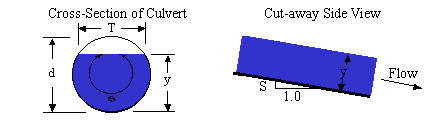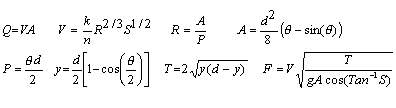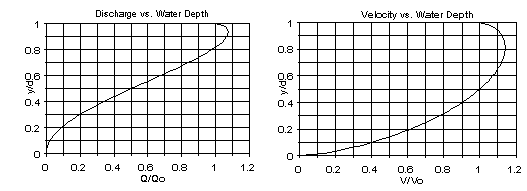Circular Culvert Design & Analysis

Compute diameter, slope, discharge, velocity, depth, Manning coeff, Froude number

Register to enable "Calculate" button.

 Select calculation, units: www.LMNOeng.com Enter n, S, d, y Enter n, S, d, T (culvert ≤ half full) Enter n, S, d, T (culvert > half full) Enter Q, n, S, d Enter Q, n, S, y/d Enter Q, n, y, d Enter Q, S, y, d Enter V, n, S, d Enter V, n, S, y/d Enter V, n, y, d Enter V, S, y, d © 2014 LMNO Engineering, Research, & Software, Ltd. Culvert Discharge, Q: m3/s ft3/s or cfs gal/min (U.S.) gal/day (U.S.) Acre-ft/yr Velocity, V: m/s ft/s Water Depth, y: m cm ft inch Culvert Diameter, d: m cm ft inch Ratio y/d: Top Width, T: m cm ft inch Manning Roughness, n: Culvert Slope, S: m/m Area, A: m2 ft2 Wetted Perimeter, P: m ft Hydraulic Radius, R: m Angle, θ: degrees Froude Number, F:

Units in circular culvert design & analysis calculator: cm=centimeter, cfs=cubic foot per second, ft=foot, gal=gallon (U.S.), m=meter, min=minute, s=second, yr=year

Circular Culvert Drawings and EquationsIntroduction to Culvert Design and Analysis using Manning Equation
The equation beginning V=.... is called the Manning Equation. It is a semi-empirical equation and is the most commonly used equation for uniform steady state flow of water in open channels (see Discussion and References for Open Channel Flow for further discussion), including culverts that are not flowing full. Because it is empirical, the Manning equation has inconsistent units which are handled through the conversion factor k. Uniform flow means that the water surface in the culvert has the same slope as the culvert itself. Uniform flow is actually only achieved in culverts that are long and have an unchanging cross-section. However, the Manning equation is used in other situations despite not strictly achieving these conditions. In situations where culvert inlet effects are important, please see our Culvert Design Calculation using Inlet and Outlet Control.

In our circular culvert design and analysis calculation, most of the combinations of inputs have analytic (closed form) solutions to compute the unknown variables; however, two require numerical solutions ("Enter Q, n, S, d" and "Enter V, n, S, d"). Our numerical solutions utilize a cubic solver that finds roots of the equations with the result accurate to at least eight significant digits. All of our software utilizes double precision internally but output is to eight significant digits.

It is possible to get two answers in our culvert calculation using "Enter Q,n,S,d" or "Enter V,n,S,d". This is because maximum Q and V do not occur when the pipe is full. Qmax occurs when y/d=0.938. If y/d is more than that, Q actually decreases due to friction. Given a pipe with diameter d, roughness n, and slope S, let Qo be the discharge when the pipe is flowing full (y/d=1). As seen on the graph below for a circular culvert, discharge is also equal to Qo when y/d=0.82. If the entered Q is greater than Qo (but less than Qmax), there will be two solution values of y/d, one between 0.82 and 0.938, and the other between 0.938 and 1. The same argument applies to V, except that Vo occurs at y/d=0.5, and Vmax occurs at y/d=0.81. If the entered V is greater than Vo (but less than Vmax), there will be two solution values of y/d, one between 0.5 and 0.81, and the other between 0.81 and 1. For further information, see Chow (1959, p. 134).

The following graphs are valid for any circular culvert roughness (n) and slope (S):
Qo=full pipe discharge; Vo=full pipe velocity:Variables in Culvert Equations [L] indicates length units. [T] indicates time units.                     To top of page
A = Flow cross-sectional area in culvert, determined normal (perpendicular) to the bottom surface [L2].
d = Culvert diameter [L].
F = Froude number. F is a non-dimensional parameter indicating the relative effect of inertial effects to gravity effects. Flow with F<1 are low velocity flows called subcritical. F>1 are high velocity flows called supercritical. Subcritical flows are controlled by downstream obstructions while supercritical flows are affected by upstream controls. F=1 flows are called critical.
g = acceleration due to gravity = 32.174 ft/s2 = 9.8066 m/s2. g is used in the equation for Froude number.
k = unit conversion factor = 1.49 if English units = 1.0 if metric units. Our culvert software converts all inputs to SI units (meters and seconds), performs the computations using k=1.0, then converts the computed quantities to units specified by the user.
n = Manning coefficient. n is a function of the culvert material, such as plastic, concrete, brick, etc. Values for n can be found in the table below of Manning's n coefficients.
P = Wetted perimeter of culvert [L]. P is the contact length (in the cross-section) between the water and the culvert.
Q = Discharge or flow rate through culvert [L3/T].
R = Culvert hydraulic radius of the flow cross-section [L].
S = Slope of culvert bottom or water surface [L/L]. Vertical distance divided by horizontal distance.
T = Top width of the flowing water in culvert [L].
V = Average velocity of the water in culvert [L/T].
y = Water depth in culvert measured normal (perpendicular) to the bottom of the culvert [L]. If the culvert has a small slope (S), then entering the vertical depth introduces only minimal error.
θ = Angle representing how full the culvert is [radians]. A culvert with θ=0 radians (0o) contains no water, a culvert with θ=π radians (180o) is half full, and a culvert with θ=2π radians (360o) is completely full.

Manning's n Coefficients                     To top of page
The table shows the Manning n values for materials most commonly used for culverts. These values were compiled from the references listed under Discussion and References and in the references at the bottom of this web page (note the footnotes which refer to specific references). A more complete table of Manning n values can be found on our Manning n page.

 Culvert Material Manning n Culvert Material Manning n Metals: Brass 0.011 Smooth Steel 0.012 Cast Iron 0.013 Corrugated Metal 0.022 Non-Metals: Corrugated Polyethylene (PE) culvert with smooth inner walls a,b 0.009-0.015 Corrugated Polyethylene (PE) culvert with corrugated inner walls c 0.018-0.025 Polyvinyl Chloride (PVC) culvert with smooth inner walls d,e 0.009-0.011 Glass 0.010 Finished Concrete 0.012 Clay Tile 0.014 Unfinished Concrete 0.014 Brickwork 0.015 Gravel 0.029 Asphalt 0.016 Earth 0.025 Masonry 0.025 Planed Wood 0.012 Unplaned Wood 0.013

Error Messages for Circular Culvert Design and Analysis Calculation                      To top of page
"Infeasible Input. T/d > 1",  "An input is ≤ 0",  "Infeasible Input. T ≤ 0",  "Infeasible Input. y/d ≥ 1".

"Invalid input". Usually appears when letters or unusual characters are entered.
"Infeasible Input". Combinations of input are unrealistic.
NaN in a numerical field means Not a Number and could be due to dividing by zero. Check input to make sure input values are reasonable.

References in Culvert Design and Analysis Calculation            To top of page
a Barfuss, Steven and J. Paul Tullis. Friction factor test on high density polyethylene pipe. Hydraulics Report No. 208. Utah Water Research Laboratory, Utah State University. Logan, Utah. 1988.

c Barfuss, Steven and J. Paul Tullis. Friction factor test on high density polyethylene pipe. Hydraulics Report No. 208. Utah Water Research Laboratory, Utah State University. Logan, Utah. 1994.

e Bishop, R.R. and R.W. Jeppson. Hydraulic characteristics of PVC sewer pipe in sanitary sewers. Utah State University. Logan, Utah. September 1975.

Chow, V. T. 1959. Open-Channel Hydraulics. McGraw-Hill, Inc.

d Neale, L.C. and R.E. Price. Flow characteristics of PVC sewer pipe. Journal of the Sanitary Engineering Division, Div. Proc 90SA3, ASCE. pp. 109-129. 1964.

b Tullis, J. Paul, R.K. Watkins, and S. L. Barfuss. Innovative new drainage pipe. Proceedings of the International Conference on Pipeline Design and Installation, ASCE. March 25-27, 1990.

LMNO Engineering, Research, and Software, Ltd.
7860 Angel Ridge Rd.   Athens, Ohio 45701  USA   Phone: (740) 592-1890
LMNO@LMNOeng.com    http://www.LMNOeng.com

To:

Culvert Design using Inlet and Outlet Conrol

Trapezoidal Channel Design

Rectangular Channels

Critical Depth in Circular Culvert

Circular culvert flow measurement using end depth in free-flow

Hydraulic jump in a circular culvert

Unit Conversions

Register